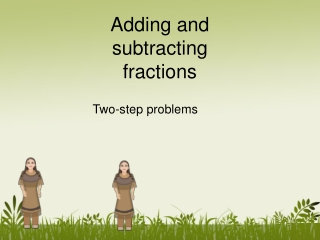DownloadDownload PresentationDownload Presentation- - - - - - - - - - - - - - - - - - - - - - - - - - - E N D - - - - - - - - - - - - - - - - - - - - - - - - - - -
##### Presentation Transcript

1. Adding and subtracting fractions Two-step problems

2. What is a two-step problem? Just how it sounds

3. Two-step problem • Solve the problem in the first step • Solve the problem in the second step • Now, you’re done

4. Here’s an example

5. The first granddaughter gathers rice from 4/7 of the shore The second granddaughter gathers rice from 2/7 of the shore How much of the shore is left?

6. What is the first step? Figure out how much was already gathered

7. I gathered 4/7 of the rice

8. I gathered 4/7 of the rice I gathered 2/7 of the rice

9. STEP ONE 4 + 2 = 6 7 7 7 This is how much rice was gathered already

10. Step two Subtract Subtract from what?

11. Step two Subtract Subtract from the whole Subtract from what?

12. This can be a tiny bit tricky You have gathered rice from this fraction 6 7 You subtract from the whole. A whole of anything – a whole lake shore, a whole pot of stew, a whole snake, a whole anything is 1.

13. 1 - 6 = 7 7 - 6 = 1 7 7 7

14. You could just count how many pieces and then see how many pieces are left 1 1 2 3 4 5 6 7 7

15. That works IF you have a model What if you don’t? 1 1 2 3 4 5 6 7 7

16. If you don’t have a model • Add up the different fractions to find the total amount you have gathered • Subtract the answer you found in step one from 1 • Hint: You might find it easier to change 1 to a fraction like 7/7